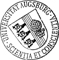## Motion of a Droplet for the Mass-Conserving Stochastic Allen-Cahn Equation

• We study the stochastic mass-conserving Allen-Cahn equation posed on a bounded two-dimensional domain with additive spatially smooth space-time noise. This equation associated with a small positive parameter describes the stochastic motion of a small almost semicircular droplet attached to domain's boundary and moving towards a point of locally maximum curvature. We apply Ito calculus to derive the stochastic dynamics of the droplet by utilizing the approximately invariant manifold introduced by Alikakos, Chen and Fusco for the deterministic problem. In the stochastic case depending on the scaling, the motion is driven by the change in the curvature of the boundary and the stochastic forcing. Moreover, under the assumption of a sufficiently small noise strength, we establish stochastic stability of a neighborhood of the manifold of droplets in L^2 and H^1, which means that with overwhelming probability the solution stays close to the manifold for very long time-scales.

Author: Dimitra C. Antonopoulou, Peter W. Bates, Dirk BlömkerORCiDGND, Georgia D. Karali urn:nbn:de:bvb:384-opus4-29654 https://opus.bibliothek.uni-augsburg.de/opus4/2965 Preprints des Instituts für Mathematik der Universität Augsburg (2015-02) Preprint English 2015 Universität Augsburg 2015/01/22 Ito calculusstochastic Allen-Cahn; mass conservation; droplet's motion; additive noise; invariant manifold; stochastic dynamics; stochastic stability Ito-Formel; Invariante Mannigfaltigkeit; Stabilität; Rauschen; Stochastisches dynamisches System `Erschienen in SIAM Journal on Mathematical Analysis, 48, 1, S. 670-708, https://doi.org/10.1137/151005105` Mathematisch-Naturwissenschaftlich-Technische Fakultät Mathematisch-Naturwissenschaftlich-Technische Fakultät / Institut für Mathematik 5 Naturwissenschaften und Mathematik / 51 Mathematik / 510 MathematikDeutsches Urheberrecht mit Print on Demand